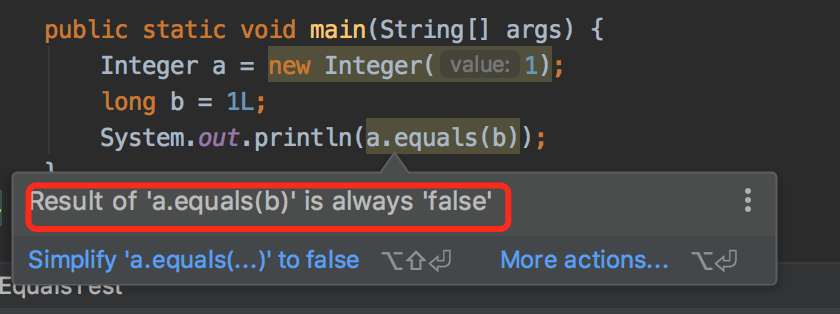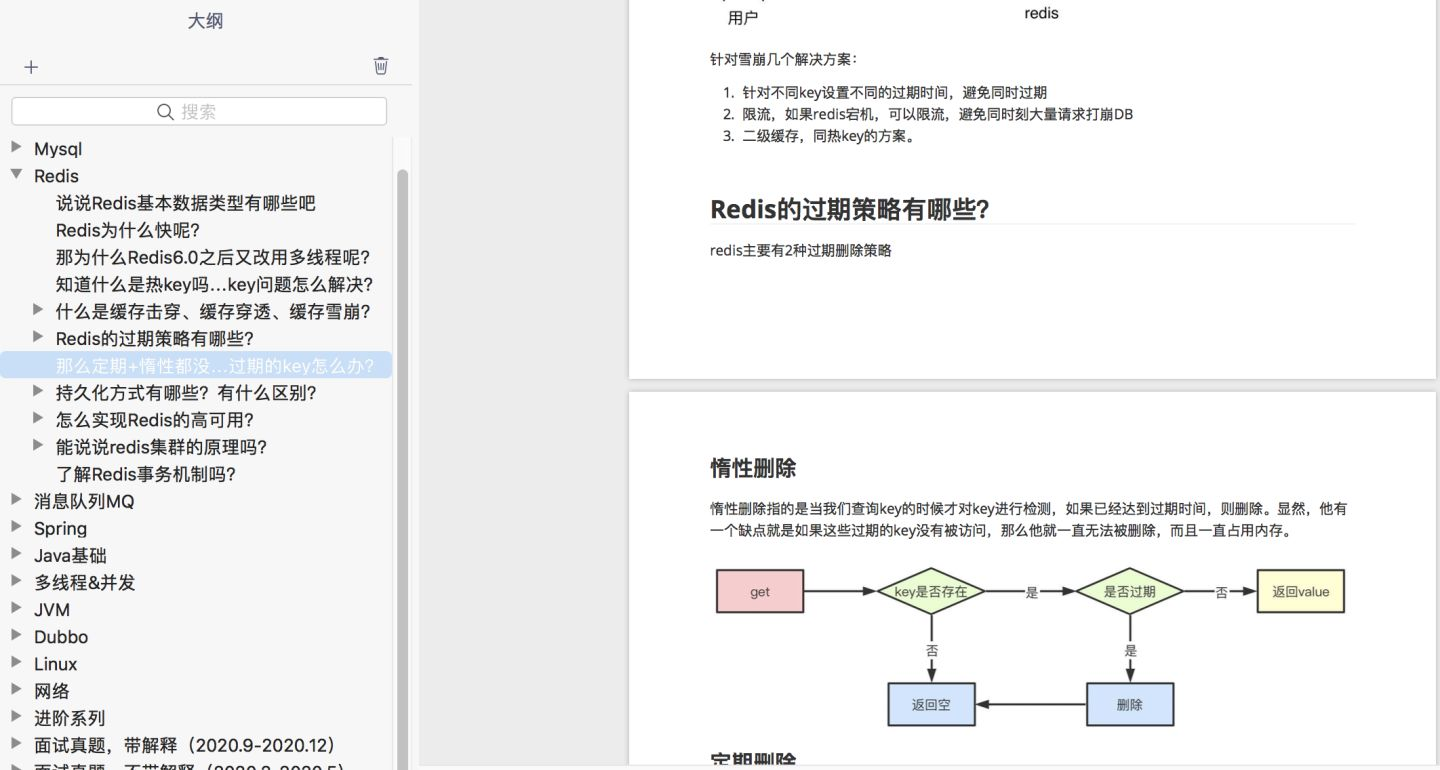# Objects.equals有坑

## 1. 案发现场

``````UserInfo userInfo = CurrentUser.getUserInfo();

if(Objects.isNull(userInfo)) {
log.info("请先登录");
return;
}

if(Objects.equals(userInfo.getId(),888)) {
sendEmail(userInfo):
}
``````

• 如果等于888，则发送邮件。
• 如果不等于888，则啥事也不干。

``````@Data
public class UserInfo {
private Long id;
private String name;
private Integer age;
}
``````

## 2. 判断相等的方法

### 2.1 使用==号

``````int a = 1;
int b = 1;
byte c = 1;
Integer d1 = new Integer(1);
Integer d2 = new Integer(1);
System.out.println(a == b);
//结果：true
System.out.println(a == c);
//结果：true
System.out.println(a == d1);
//结果：true
System.out.println(d2 == a);
//结果：true
System.out.println(d1 == d2);
//结果：false
``````

Integer和int比较时，会自动拆箱，这是比较值是否相等。

``````Integer d3 = 1;
Integer d4 = 1;
Integer d5 = 128;
Integer d6 = 128;
System.out.println(d3 == d4);
//结果：true
System.out.println(d5 == d6);
//结果：false
``````

``````String e = "abc";
String f = "abc";
String g = new String("abc");
String h = new String("abc");
System.out.println(e == f);
//结果：true
System.out.println(e == g);
//结果：false
System.out.println(g == h);
//结果：false
``````

### 2.2 使用equals方法

``````String g = new String("abc");
String h = new String("abc");
System.out.println(g == h);
//结果：false
``````

equals方法其实是Object类中的方法：

``````public boolean equals(Object obj) {
return (this == obj);
}
``````

``````public boolean equals(Object anObject) {
if (this == anObject) {
return true;
}
if (anObject instanceof String) {
String anotherString = (String)anObject;
int n = value.length;
if (n == anotherString.value.length) {
char v1[] = value;
char v2[] = anotherString.value;
int i = 0;
while (n-- != 0) {
if (v1[i] != v2[i])
return false;
i++;
}
return true;
}
}
return false;
}
``````

nice，这样就能解决g和h判断的问题：

``````String e = "abc";
String f = "abc";
System.out.println(e.equals(f));
//结果：true
``````

## 3. 空指针异常

``````int a = 1;
Integer b = new Integer(1);
Integer c = null;
System.out.println(a == b);
//结果：true
System.out.println(a == c);
//结果：NullPointerException
``````

int和Integer使用==号判断是否相等时，Integer会自动拆箱成int。

``````String e = null;
String f = "abc";
System.out.println(e.equals(f));
//结果：NullPointerException
``````

``````String e = null;
String f = "abc";
System.out.println(equals(e, f));
``````

``````private static boolean equals(String e, String f) {
if (e == null) {
return f == null;
}
return e.equals(f);
}
``````

## 4. Objects.equals的作用

`Objects`类位于`java.util`包下，它是里面提供了很多对象操作的辅助方法。

``````public static boolean equals(Object a, Object b) {
return (a == b) || (a != null && a.equals(b));
}
``````

equals方法的判断逻辑如下：

1. 该方法先判断对象a和b的引用是否相等，如果相等则直接返回true。
2. 如果引用不相等，则判断a是否为空，如果a为空则返回false。
3. 如果a不为空，调用对象的equals方法进一步判断值是否相等。

``````int a = 1;
int b = 1;
Integer c = null;

System.out.println(Objects.equals(a, c));
//结果：false
System.out.println(Objects.equals(c, a));
//结果：false
System.out.println(Objects.equals(a, b));
//结果：true
``````

``````int a = 1;
Integer c = null;
System.out.println(equals(a, c));
//结果：false
``````

``````private static boolean equals(Object a, Object b) {
return a == b;
}
``````

## 5. Objects.equals的坑

``````Integer a = 1;
long b = 1L;
System.out.println(Objects.equals(a, b));
//结果：false
``````

``````Integer a = 1;
long b = 1L;
System.out.println(a == b);
//结果：true
``````

a和b明明都是1，为什么使用Objects.equals方法判断不相等呢？

``````public boolean equals(Object obj) {
if (obj instanceof Integer) {
return value == ((Integer)obj).intValue();
}
return false;
}
``````

``````Integer a = 1;
long b = 1L;
System.out.println(Objects.equals(b, a));
//结果：false
``````

``````public boolean equals(Object obj) {
if (obj instanceof Long) {
return value == ((Long)obj).longValue();
}
return false;
}
``````

``````Integer a = 1;
long b = 1L;
System.out.println(Objects.equals(a, (int)b));
//结果：true
``````

``````Integer a = 1;
long b = 1L;
System.out.println(Objects.equals(b, (long)a));
//结果：true
``````

``````Integer a = 1;
long b = 1L;
System.out.println(a==b);
//结果：true
``````

``````Integer a = new Integer(1);
long b = 1L;
System.out.println(a.equals(b));
``````1. Long类型和Integer类型比较，比如：用户id的场景。
2. Byte类型和Integer类型比较，比如：状态判断的场景。
3. Double类型和Integer类型比较，比如：金额为0的判断场景。